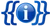Template documentation (for the template shown above, sometimes hidden or invisible)

## ContentsDocumentation

## Usage

A border of 2px (1px width on each side) corresponds to a 5%. Therefore, with a 2px border — like on Encyclopedia Gamia:Tutorial (Wrap-up and more info) — the width needs to be 95% for the table to fit within the screen. Since it's possible that some tables might not have a border, correction on the individual page could be made after substitution. Another way do a two column table is like this:

 ```{{Col-begin|width=95%}} |- | Left column | Right column |} ```

Template:Col-1-of-3`{{Col-begin}}``{{Col-1-of-2}}` Column 1 here `{{Col-2-of-2}}` Column 2 here `{{Col-end}}`Template:Col-2-of-3 (Template talk) `{{Col-1-of-3}}` Column 1 here `{{Col-2-of-3}}` Column 2 here `{{Col-3-of-3}}` Column 3 here `{{Col-end}}`Template:Col-3-of-3`{{Col-begin}}``{{Col-1-of-4}}` Column 1 here `{{Col-2-of-4}}` Column 2 here `{{Col-3-of-4}}` Column 3 here `{{Col-4-of-4}}` Column 4 here `{{Col-end}}`

Template:Col-1-of-3 {{Col-begin|width=}} `{{Col-1-of-5}}` Column 1 here `{{Col-2-of-5}}` Column 2 here `{{Col-3-of-5}}` Column 3 here `{{Col-4-of-5}}` Column 4 here `{{Col-5-of-5}}` Column 5 here `{{Col-end}}`Template:Col-2-of-3 {{Col-begin|bgColor=transparent}} `{{Col-1-of-6}}` Column 1 here `{{Col-2-of-6}}` Column 2 here `{{Col-3-of-6}}` Column 3 here `{{Col-4-of-6}}` Column 4 here `{{Col-5-of-6}}` Column 5 here `{{Col-6-of-6}}` Column 6 here `{{Col-end}}`Template:Col-3-of-3 {{Col-begin|class=references-small}} `{{Col-break}}` Column 1 here `{{Col-break}}` Column 2 here `{{Col-break}}` Column 3 here `{{Col-break}}` Column 4 here `{{Col-break}}` Column 5 here `{{Col-break}}` Column 6 here `{{Col-end}}`

Template:Col-1-of-3`{{Top}}` Column 1 here `{{Mid}}` Column 2 here `{{Bottom}}`Template:Col-2-of-3 {{Columns-start|num=3}} Column 1 here `{{Column}}` Column 2 here `{{Column}}` Column 3 here `{{Columns-end}}`Template:Col-3-of-3`{{Multicol}}` Column 1 here `{{Multicol-break}}` Column 2 here `{{Multicol-break}}` Column 3 here `{{Multicol-break}}` Column 4 here `{{Multicol-end}}`

• `{{Col-begin}}` (`{{Col-start}}`), `{{Col-begin-small}}` links talk view
• `{{Col-2}}` (`{{Col-1-of-2}}`, `{{Col-2-of-2}}`)
• `{{Col-3}}` (`{{Col-1-of-3}}`, `{{Col-2-of-3}}`, `{{Col-3-of-3}}`)
• `{{Col-4}}` (`{{Col-1-of-4}}`, `{{Col-2-of-4}}`, `{{Col-3-of-4}}`, `{{Col-4-of-4}}`)
• `{{Col-5}}` (`{{Col-1-of-5}}`, `{{Col-2-of-5}}`, `{{Col-3-of-5}}`, `{{Col-4-of-5}}`, `{{Col-5-of-5}}`)
• `{{Col-6}}` (`{{Col-1-of-6}}`, `{{Col-2-of-6}}`, `{{Col-3-of-6}}`, `{{Col-4-of-6}}`, `{{Col-5-of-6}}`, `{{Col-6-of-6}}`)
• `{{Col-break}}`
• `{{Col-end}}` (`{{End}}`)
• `{{Top}}`, `{{Mid}}`, `{{Bottom}}`
• `{{Columns-start}}`, `{{Column}}`, `{{Columns-end}}` links talk view
• `{{Multicol}}`, `{{Multicol-break}}`, `{{Multicol-end}}` links talk view

Visit Template:Col-begin/doc to edit this text! (How does this work?)